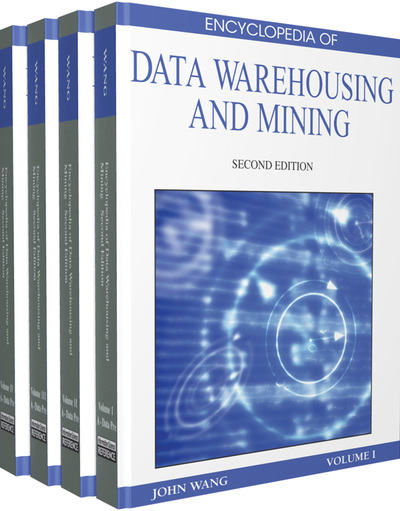# Multiple Hypothesis Testing for Data Mining

Sach Mukherjee (University of Oxford, UK)
DOI: 10.4018/978-1-60566-010-3.ch215

## Abstract

A number of important problems in data mining can be usefully addressed within the framework of statistical hypothesis testing. However, while the conventional treatment of statistical significance deals with error probabilities at the level of a single variable, practical data mining tasks tend to involve thousands, if not millions, of variables. This Chapter looks at some of the issues that arise in the application of hypothesis tests to multi-variable data mining problems, and describes two computationally efficient procedures by which these issues can be addressed.
Chapter Preview
Top

## Background

Many problems in commercial and scientific data mining involve selecting objects of interest from large datasets on the basis of numerical relevance scores (“object selection”). This Section looks briefly at the role played by hypothesis tests in problems of this kind. We start by examining the relationship between relevance scores, statistical errors and the testing of hypotheses in the context of two illustrative data mining tasks. Readers familiar with conventional hypothesis testing may wish to progress directly to the main part of the Chapter.

As a topical example, consider the differential analysis of gene microarray data (Piatetsky-Shapiro & Tamayo, 2004; Cui & Churchill, 2003). The data consist of expression levels (roughly speaking, levels of activity) for each of thousands of genes across two or more conditions (such as healthy and diseased). The data mining task is to find a set of genes which are differentially expressed between the conditions, and therefore likely to be relevant to the disease or biological process under investigation. A suitably defined mathematical function (the t-statistic is a canonical choice) is used to assign a “relevance score” to each gene and a subset of genes selected on the basis of the scores. Here, the objects being selected are genes.

As a second example, consider the mining of sales records. The aim might be, for instance, to focus marketing efforts on a subset of customers, based on some property of their buying behavior. A suitably defined function would be used to score each customer by relevance, on the basis of his or her records. A set of customers with high relevance scores would then be selected as targets for marketing activity. In this example, the objects are customers.

Clearly, both tasks are similar; each can be thought of as comprising the assignment of a suitably defined relevance score to each object and the subsequent selection of a set of objects on the basis of the scores. The selection of objects thus requires the imposition of a threshold or cut-off on the relevance score, such that objects scoring higher than the threshold are returned as relevant. Consider the microarray example described above. Suppose the function used to rank genes is simply the difference between mean expression levels in the two classes. Then the question of setting a threshold amounts to asking how large a difference is sufficient to consider a gene relevant. Suppose we decide that a difference in means exceeding x is ‘large enough’: we would then consider each gene in turn, and select it as “relevant” if its relevance score equals or exceeds x. Now, an important point is that the data are random variables, so that if measurements were collected again from the same biological system, the actual values obtained for each gene might differ from those in the particular dataset being analyzed. As a consequence of this variability, there will be a real possibility of obtaining scores in excess of x from genes which are in fact not relevant.

## Complete Chapter List

Search this Book:
Reset